# Introduction to the bSims package

## Introduction

The bSims R package is a highly scientific and utterly addictive bird point count simulator. Highly scientific, because it implements a spatially explicit mechanistic simulation that is based on statistical models widely used in bird point count analysis (i.e. removal models, distance sampling), and utterly addictive because the implementation is designed to allow rapid interactive exploration (via shiny apps) and efficient simulation (supporting various parallel backends), thus elevating the user experience.

The goals of the package are to:

1. allow easy testing of statistical assumptions and explore effects of violating these assumptions,
2. aid survey design by comparing different options,
3. and most importantly, to have fun while doing it via an intuitive and interactive user interface.

The simulation interface was designed with the following principles in mind:

1. isolation: the spatial scale is small (local point count scale) so that we can treat individual landscapes as more or less homogeneous units (but see below how certain stratified designs and edge effects can be incorporated) and independent independent in space and time;
2. realism: the implementation of biological mechanisms and observation processes are realistic, defaults are chosen to reflect common practice and assumptions;
3. efficiency: implementation is computationally efficient utilizing parallel computing backends when available;
4. extensibility: the package functionality is well documented and easily extensible.

This documents outlines the major functionality of the package. First we describe the motivation for the simulation and the details of the layers. Then we outline an interactive workflow to design simulation studies and describe how to run efficient simulation experiments. Finally we present some of the current limitations of the framework and how to extend the existing functionality of the package to incorporate more of the biological realism into the simulations.

## Simulation layers

Introductory stats books begin with the coin flip to introduce the binomial distribution. In R we can easily simulate an outcome from such a random variable $$Y \sim Binomial(1, p)$$ doing something like this:

p <- 0.5

Y <- rbinom(1, size = 1, prob = p)

But a coin flip in reality is a lot more complicated: we might consider the initial force, the height of the toss, the spin, and the weight of the coin.

Bird behavior combined with the observation process presents a more complicated system, that is often treated as a mixture of a count distribution and a detection/nondetection process, e.g.:

D <- 2 # individuals / unit area
A <- 1 # area
p <- 0.8 # probability of availability given presence
q <- 0.5 # probability of detection given availability

N <- rpois(1, lambda = A * D)
Y <- rbinom(1, size = N, prob = p * q)

This looks not too complicated, corresponding to the true abundance being a random variables $$N \sim Poisson(DA)$$, while the observed count being $$Y \sim Binomial(N, pq)$$. This is the exact simulation that we need when we want to make sure that an estimator is capable of estimating the model parameters (lambda and prob here). But such probabilistic simulations are not very useful when we are interested how well the model captures important aspects of reality.

Going back to the Poisson–Binomial example, N would be a result of all the factors influencing bird abundance, such as geographical location, season, habitat suitability, number of conspecifics, competitors, or predators. Y however would largely depend on how the birds behave depending on timing, or how an observer might detect or miss the different individuals, or count the same individual twice, etc.

Therefore the package has layers, that by default are conditionally independent of each other. This design decision is meant to facilitate the comparison of certain settings while keeping all the underlying realizations identical, thus helping to pinpoint effects without the extra variability introduced by all the other effects.

The conditionally independent layers of a bSims realization are the following, with the corresponding function:

1. landscape (bsims_init),
2. population (bsims_populate),
3. behavior with movement and vocalization events (bsims_animate),
4. the physical side of the observation process (bsims_detect), and
5. the human aspect of the observation process (bsims_transcribe).

See this example as a sneak peek that we’ll explain in the following subsections:

library(bSims)
#> Loading required package: intrval
#> Loading required package: mefa4
#> Loading required package: Matrix
#> mefa4 0.3-6   2019-06-20
#> Loading required package: MASS
#> Loading required package: deldir
#> deldir 0.1-23
#> bSims 0.2-1   2019-12-16      woonk-a-chunk

phi <- 0.5                 # singing rate
tau <- 1:3                 # detection distances by strata
tbr <- c(3, 5, 10)         # time intervals
rbr <- c(0.5, 1, 1.5, Inf) # count radii

l <- bsims_init(10, 0.5, 1)# landscape
p <- bsims_populate(l, 1)  # population
e <- bsims_animate(p,      # events
vocal_rate=phi)
d <- bsims_detect(e,       # detections
tau=tau)
x <- bsims_transcribe(d,   # transcription
tint=tbr, rint=rbr)

get_table(x) # removal table
#>          0-3min 3-5min 5-10min
#> 0-50m         1      0       1
#> 50-100m       3      0       0
#> 100-150m      1      0       0
#> 150+m         8      2       0
plot(x)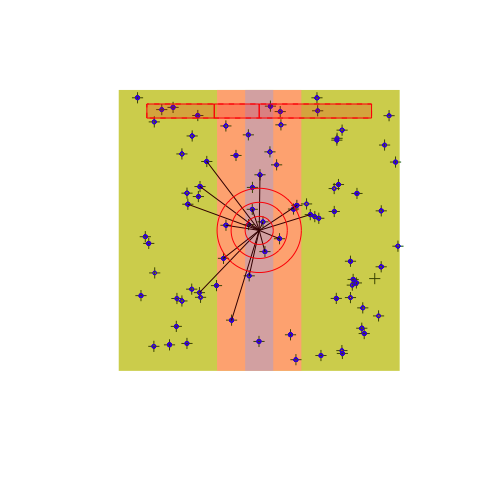### Landscape

The bsims_ini function sets up the geometry of a local landscape. The extent of the landscape determines the edge lengths of a square shaped area. With no argument values passed, the function assumes a homogeneous habitat (H) in a 10 units x 10 units landscape, 1 unit is 100 meters. Having units this way allows easier conversion to ha as area unit that is often used in the North American bird literature. As a result, our landscape has an area of 1 km$$^2$$.

The road argument defines the half-width of the road that is placed in a vertical position. The edge argument defines the width of the edge stratum on both sides of the road. Habitat (H), edge (E), and road (R) defines the 3 strata that we refer to by their initials (H for no stratification, HER for all 3 strata present).

The origin of the Cartesian coordinate system inside the landscape is centered at the middle of the square. The offset argument allows the road and edge strata to be shifted to the left (negative values) or to the right (positive values) of the horizontal axis. This makes it possible to create landscapes with only two strata. The bsims_init function returns a landscape object (with class ‘bsims_landscape’).

(l1 <- bsims_init(extent = 10, road = 0, edge = 0, offset = 0))
#> bSims landscape
#>   1 km x 1 km
#>   stratification: H
(l2 <- bsims_init(extent = 10, road = 1, edge = 0, offset = 0))
#> bSims landscape
#>   1 km x 1 km
#>   stratification: HR
(l3 <- bsims_init(extent = 10, road = 0.5, edge = 1, offset = 2))
#> bSims landscape
#>   1 km x 1 km
#>   stratification: HER
(l4 <- bsims_init(extent = 10, road = 0, edge = 5, offset = 5))
#> bSims landscape
#>   1 km x 1 km
#>   stratification: HE

op <- par(mfrow = c(2, 2))
plot(l1, main = "Habitat")
points(0, 0, pch=3)
plot(l2, main = "Habitat & road")
lines(c(0, 0), c(-5, 5), lty=2)
plot(l3, main = "Habitat, edge, road + offset")
arrows(0, 0, 2, 0, 0.1, 20)
lines(c(2, 2), c(-5, 5), lty=2)
points(0, 0, pch=3)
plot(l4, main = "2 habitats")
arrows(0, 0, 5, 0, 0.1, 20)
lines(c(5, 5), c(-5, 5), lty=2)
points(0, 0, pch=3)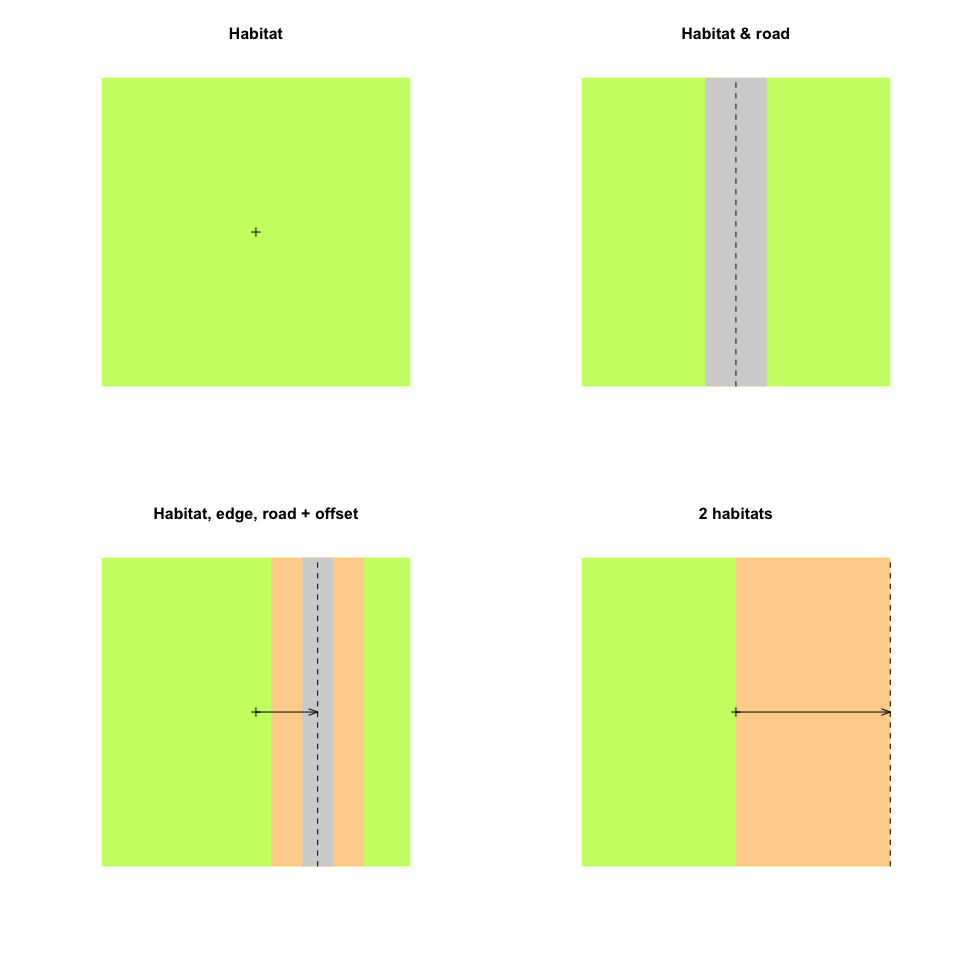par(op)

### Population

The bsims_populate function populates the landscape we created by the bsims_init function, which is the first argument we have to pass to bsims_populate. The function returns a population object (with class ‘bsims_population’). The most important argument that controls how many individuals will inhabit our landscape is density that defines the expected value of individuals per unit area (1 ha). By default, density = 1 ($$D=1$$) and we have 100 ha in the landscape ($$A=100$$) which translates into 100 individuals on average ($$E[N]=\lambda=AD$$). The actual number of individuals in the landscape might deviate from this expectation, because $$N$$ is a random variable ($$N \sim f(\lambda)$$). The abund_fun argument controls this relationship between the expected ($$\lambda$$) and realized abundance ($$N$$). The default is a Poisson distribution:

bsims_populate(l1)
#> bSims population
#>   1 km x 1 km
#>   stratification: H
#>   total abundance: 100

Changing abund_fun can be useful to make abundance constant or allow under or overdispersion, e.g.:

summary(rpois(100, 100)) # Poisson variation
#>    Min. 1st Qu.  Median    Mean 3rd Qu.    Max.
#>    76.0    93.0   101.5   100.9   108.0   129.0
summary(MASS::rnegbin(100, 100, 0.8)) # NegBin variation
#>    Min. 1st Qu.  Median    Mean 3rd Qu.    Max.
#>     0.0    28.5    74.5   102.9   144.2   618.0
negbin <- function(lambda, ...) MASS::rnegbin(1, lambda, ...)
bsims_populate(l1, abund_fun = negbin, theta = 0.8)
#> bSims population
#>   1 km x 1 km
#>   stratification: H
#>   total abundance: 61
## constant abundance
bsims_populate(l1, abund_fun = function(lambda, ...) lambda)
#> bSims population
#>   1 km x 1 km
#>   stratification: H
#>   total abundance: 100

Once we determine how many individuals will populate the landscape, we have control over the spatial arrangement of the nest location for each individual. The default is a homogeneous Poisson point process (complete spatial randomness). Deviations from this can be controlled by the xy_fun. This function takes distance as its only argument and returns a numeric value between 0 and 1. A function function(d) reurn(1) would be equivalent with the Poisson process, meaning that every new random location is accepted with probability 1 irrespective of the distance between the new location and the previously generated point locations in the landscape. When this function varies with distance, it leads to a non-homogeneous point process via this accept-reject algorithm. The other arguments (margin, maxit, fail) are passed to the underlying accepreject function to remove edge effects and handle high rejection rates.

In the next example, we fix the abundance to be constant (i.e. not a random variable, $$N=\lambda$$) and different spatial point processes:

D <- 0.5
f_abund <- function(lambda, ...) lambda

## systematic
f_syst <- function(d)
(1-exp(-d^2/1^2) + dlnorm(d, 2)/dlnorm(exp(2-1),2)) / 2
## clustered
f_clust <- function(d)
exp(-d^2/1^2) + 0.5*(1-exp(-d^2/4^2))

p1 <- bsims_populate(l1, density = D, abund_fun = f_abund)
p2 <- bsims_populate(l1, density = D, abund_fun = f_abund, xy_fun = f_syst)
p3 <- bsims_populate(l1, density = D, abund_fun = f_abund, xy_fun = f_clust)

distance <- seq(0,10,0.01)
op <- par(mfrow = c(3, 2))
plot(distance, rep(1, length(distance)), type="l", ylim = c(0, 1),
main = "random", ylab=expression(f(d)), col=2)
plot(p1)

plot(distance, f_syst(distance), type="l", ylim = c(0, 1),
main = "systematic", ylab=expression(f(d)), col=2)
plot(p2)

plot(distance, f_clust(distance), type="l", ylim = c(0, 1),
main = "clustered", ylab=expression(f(d)), col=2)
plot(p3)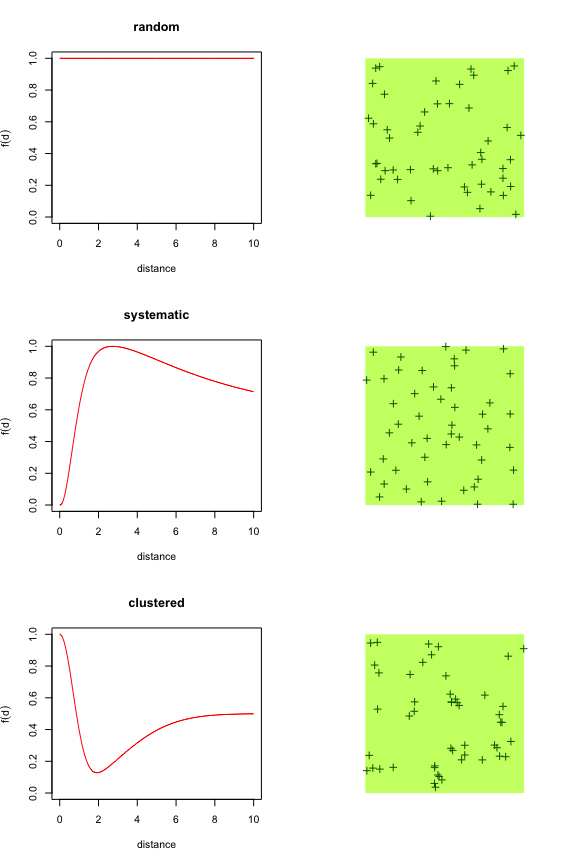par(op)

The get_nests function extracts the nest locations. get_abundance and get_density gives the total abundance ($$N$$) and density ($$D=N/A$$, where $$A$$ is extent^2) in the landscape, respectively.

If the landscape is stratified, that has no effect on density unless we specify different values through the density argument as a vector of length 3 referring to the HER strata:

D <- c(H = 2, E = 0.5, R = 0)

op <- par(mfrow = c(2, 2))
plot(bsims_populate(l1, density = D), main = "Habitat")
plot(bsims_populate(l2, density = D), main = "Habitat & road")
plot(bsims_populate(l3, density = D), main = "Habitat, edge, road + offset")
plot(bsims_populate(l4, density = D), main = "2 habitats")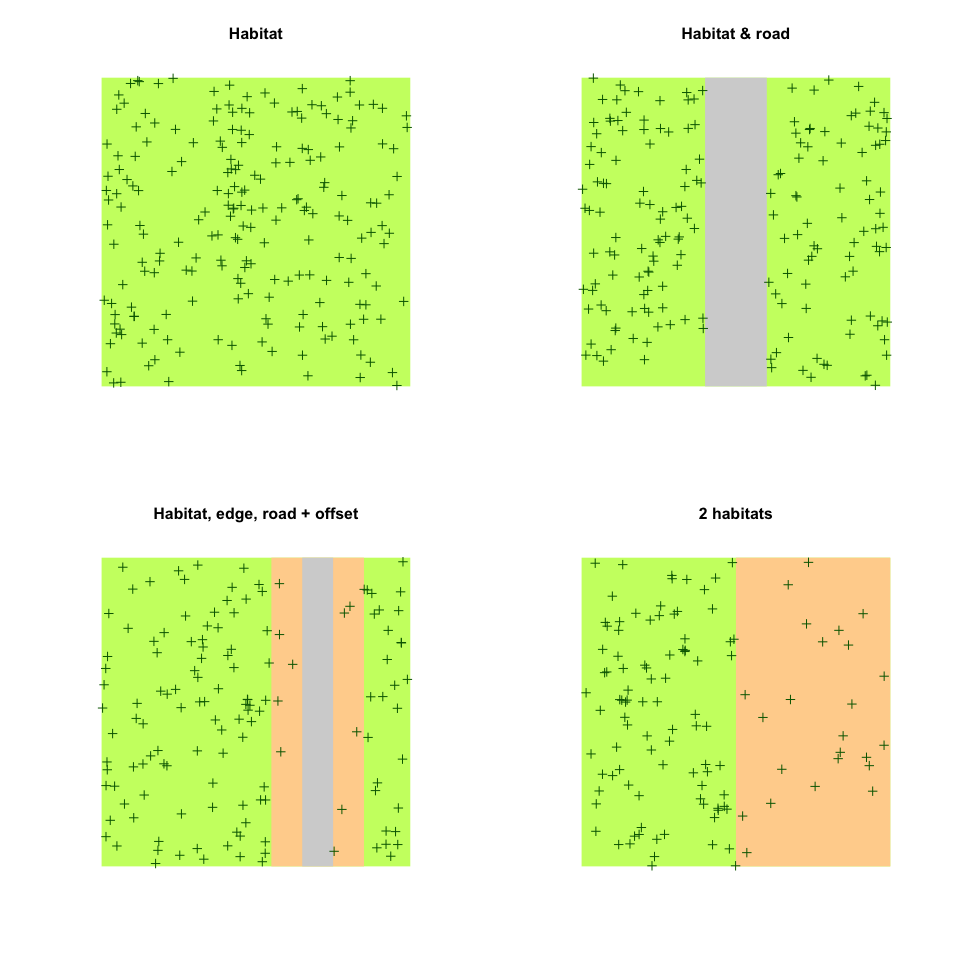par(op)

### Behavior

The bsims_animate function animates the population created by the bsims_populate function. bsims_animate returns an events object (with class ‘bsims_events’). The most important arguments are governing the duration of the simulation in minutes, the vocalization (vocal_rate), and the movement (move_rate) rates as average number of events per minute.

We can describe these behavioral events using survival modeling terminology. Event time ($$T$$) is a continuous random variable. In the simplest case, its probability density function is the Exponential distribution: $$f(t)=\phi e^{-t\phi}$$. The corresponding cumulative distribution function is: $$F(t)=\int_{0}^{t} f(t)dt=1-e^{-t\phi}=p_t$$, giving the probability that the event has occurred by duration $$t$$. The parameter $$\phi$$ is the rate of the Exponential distribution with mean $$1/\phi$$ and variance $$1/\phi^2$$.

In survival models, the complement of $$F(t)$$ is called the survival function ($$S(t)=1-F(t)$$, $$S(0)=1$$), which gives the probability that the event has not occurred by duration $$t$$. The hazard function ($$\lambda(t)=f(t)/S(t)$$) defines the instantaneous rate of occurrence of the event (risk, the density of events at $$t$$ divided by the probability of surviving). The cumulative hazard (cumulative risk) is the sum of the risks between duration 0 and $$t$$ ($$\Lambda(t)=\int_{0}^{t} \lambda(t)dt$$).

The simplest survival distribution assumes constant risk over time ($$\lambda(t)=\phi$$), which corresponds to the Exponential distribution. The Exponential distribution also happens to describe the lengths of the inter-event times in a homogeneous Poisson process (events are independent, it is a ‘memory-less’ process).

bsims_animate uses independent Exponential distributions with rates vocal_rate and move_rate to simulate vocalization and movement events, respectively. The get_events function extracts the events as a data frame with columns describing the location (x, y) and time (t) of the events (v is 1 for vocalizations and 0 otherwise) for each individual (i gives the individual identifier that links individuals to the nest locations)

l <- bsims_init()
p <- bsims_populate(l, density = 0.5)
e1 <- bsims_animate(p, vocal_rate = 1)

head(get_events(e1))
#>            x         y         t v  i
#> 1 -3.6144923 -4.763833 0.1224272 1 16
#> 2 -0.5535331 -4.942269 0.1499922 1 22
#> 3  0.5747048  2.392937 0.1654052 1 26
#> 4  3.7020017  1.818227 0.1697386 1 31
#> 5  4.4833641  1.862739 0.1902219 1 27
#> 6  4.1826974  3.908325 0.1980518 1 32
plot(get_events(e1))
curve((1-exp(-1*x)) * get_abundance(e1), col=2, add=TRUE)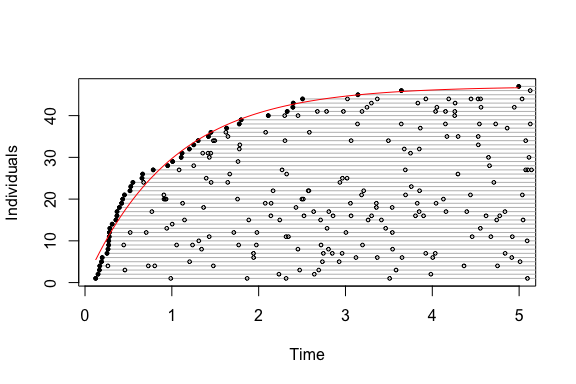There are no movement related events when move_rate = 0, the individuals are always located at the nest, i.e. there is no within territory movement. If we increase the movement rate, we also have to increase the value of movement, that is the standard deviation of bivariate Normal kernels centered around each nest location. This kernel is used to simulate new locations for the movement events.

e2 <- bsims_animate(p, move_rate = 1, movement = 0.25)

op <- par(mfrow = c(1, 2))
plot(e1, main = "Closure")
plot(e2, main = "Movement")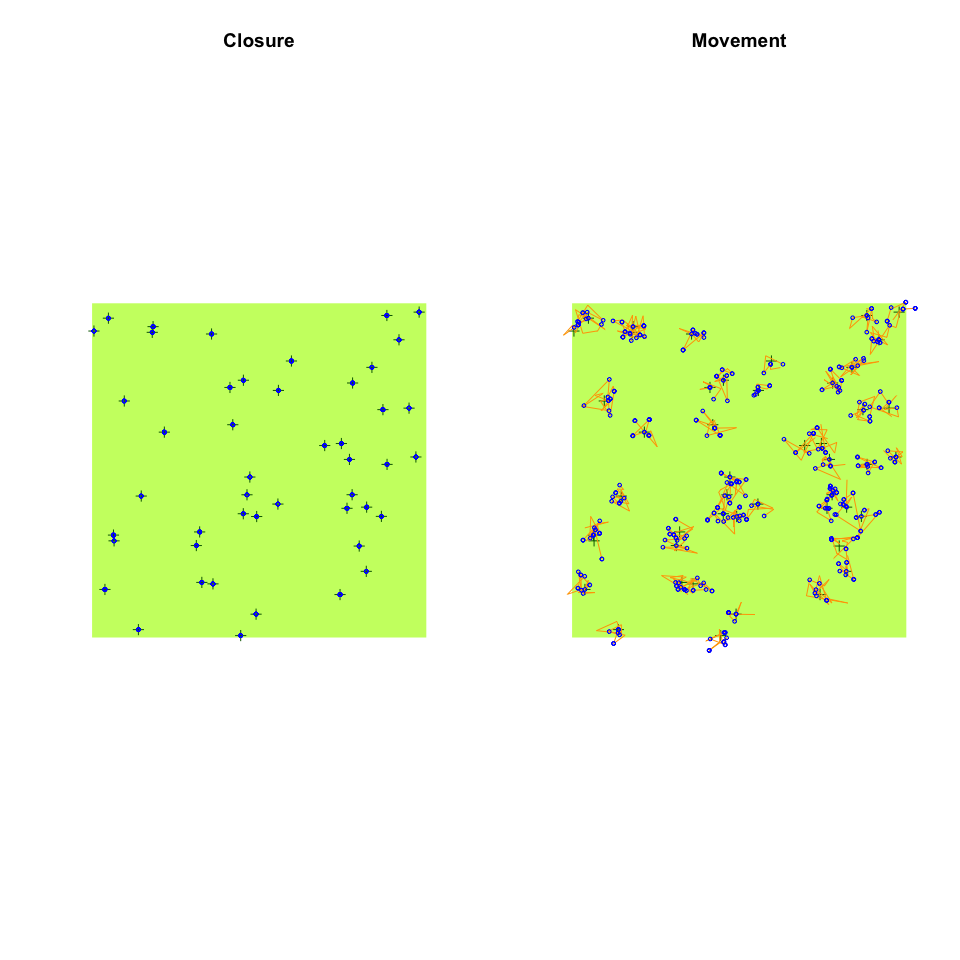par(op)

Individuals in the landscape might have different vocalization rates depending on, e.g., breeding status. Such heterogeneity can be added to the simulations as a finite mixture: vocal_rate and move_rate can be supplied as a vector, each element giving the rate for the groups. The mixture argument is then used to specify the mixture proportions.

e3 <- bsims_animate(p,
vocal_rate = c(25, 1), mixture = c(0.33, 0.67))

plot(get_events(e3))
curve((1-0.67*exp(-1*x)) * get_abundance(e3), col=2, add=TRUE)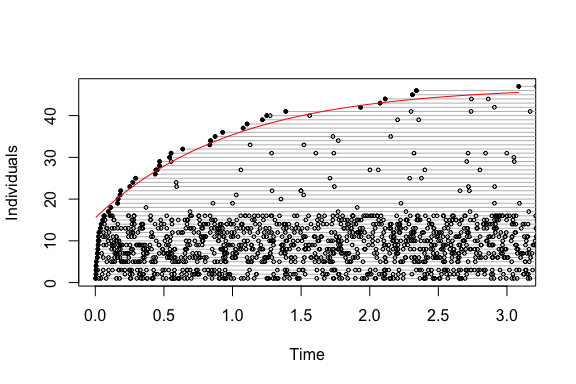Vocal and movement rates (and corresponding kernel standard deviations) can be defined four different ways:

• a single number: constant behavior patterns, no groups,
• a vector of length length(mixture): behavior based finite mixture groups,
• a vector of length 3 with mixture = 1: mixtures correspond to HER strata,
• or a matrix of dimension 3 $$\times$$ length(mixture): HER strata $$\times$$ number of behavior based groups.

Strata based groups are tracked by column s, behavior based groups are tracked as the column g in the output of get_nests.

Here is how different territory sizes can be achieved in a two-habitat landscape:

plot(bsims_animate(bsims_populate(l4, density = D),
move_rate = c(0.5, 1, 1), movement = c(0, 0.2, 0.2),
mixture = 1), main="Strata based mixtures")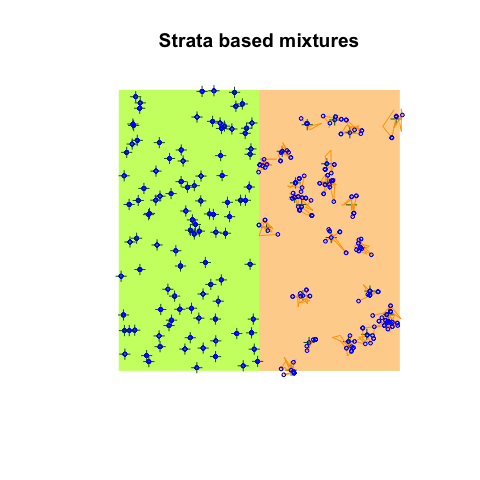Stratum related behavior groups depend on the nest location. Sometimes it makes sense to restrict movement even further, i.e. individuals do not land in certain strata (but can cross a stratum if movement is large enough). For example we can restrict movement into the road stratum (this requires density to be 0 in that stratum):

op <- par(mfrow = c(1, 2))
plot(bsims_animate(bsims_populate(l2, density = D),
move_rate = 1, movement = 0.3,
avoid = "none"), main="Movement not restricted")
plot(bsims_animate(bsims_populate(l2, density = D),
move_rate = 1, movement = 0.3,
avoid = "R"), main="Movement restricted")